# Quiz 16: Cost-Volume-Profit Analysis

In this solution we are required to calculate the variable cost, contribution margin and contribution margin ratio, hence it shall be done in following manner: 1. Here in 1 st Part we need to calculate: a. Variable product cost per unit: The variable cost per unit is a cost which keeps on changes with changes in the production levels. Hence the variable cost per unit shall be calculated in following manner: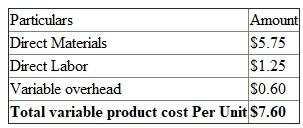b. The total variable cost per unit shall be calculated in following manner: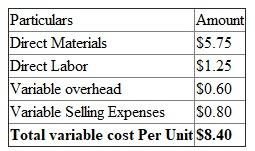c. The Contribution margin shall be calculated as a difference between Sales Price and Variable cost per unit:d. Contribution margin ratio is calculated using formula:e. The total fixed expense shall be calculated by adding up the fixed expenses of the factory and selling hence it shall be calculated in following manner: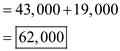2. The Contribution margin income statement is prepared by deducting the variable expenses and thereafter deducting fixed expenses: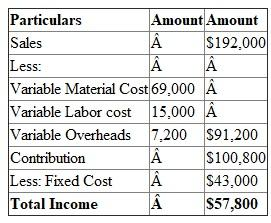3. Here in 1 st Part we need to calculate the: a. Variable product cost per unit: The variable cost per unit is a cost which keeps on changes with changes in the production levels. Hence the variable cost per unit shall be calculated in following manner: It shall remain unchanged in comparison to Part-I as variable charges are unchanged.b. The total variable cost per unit shall be calculated in following manner: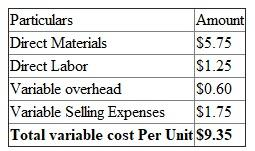c. The Contribution margin shall be calculated as a difference between Sales Price and Variable cost:d. Contribution margin ratio is calculated using formula:e. The total fixed expense shall be calculated by adding up the fixed expenses of the factory and selling hence it shall be calculated in following manner:1. The Sales Commission per unit shall be calculated in the following manner:The contribution margin is a difference between sales and variable cost, it shall be calculated in the following manner: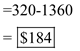While calculating variable costs the Direct Material, Direct Labor and Variable overhead is added up. 2. The breakeven point is a point where there is no profit or loss, it is calculated using formula:Note: While calculation of variable cost the Selling commission is also considered and while calculation of Fixed Expenses the Administrative and selling expenses are also considered. The income statement shall be calculated I following manner: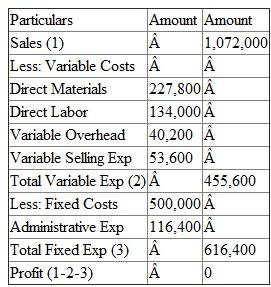Note: The income statement is for 3,350 units which is breakeven point. 3. The target profit can be calculated by using formula:4. For the target income of 322,000, it shall be calculated in following manner:Conclusion : So lesser number of units shall be sold.